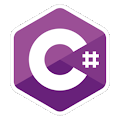Programming Course in C# ¡Free!

# Statistics

Proposed exercise

Write a program to compute several basic statistical operations: it will accept numbers from the user and show their sum, average, minimum and maximum, as in the following example:

Number? 5
Total=5 Amount=1 Average=5 Maximum=5 Minimum=5
Number? 2
Total=7 Amount=2 Average=3 Maximum=5 Minimum=2
Number? 0
Bye!

(As seen in this example, the program will finish when the user enters 0)

Output

Solution

```using System;
public class Statistics
{
public static void Main()
{
Console.Write("Enter numberber: ");

int maximum = number; int minimum = number;

int total = 0;
int amount = 0;

while ( number != 0 )
{
amount++;
total += number;

if ( number > maximum )
maximum = number;

if ( number < minimum )
minimum = number;

Console.WriteLine(
"Total={0} Amount={1} Average={2} aximum={3} minimum={4}",
total, amount, total/amount, maximum, minimum);

Console.Write("Enter numberber: ");